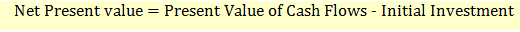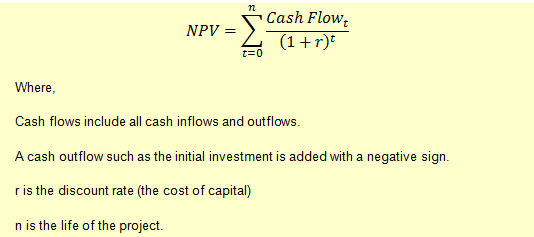# Net Present Value

The net present value is the most commonly used method to decide whether to invest in a project or not.

The net present value of a project is equal to the sum of the present value of all after-tax cash flows from the project minus the initial investment.The investment decision using the NPV method will be based on whether the NPV is positive or negative. The NPV will be positive if the present value of all future cash flows is higher than the initial investment. A positive NPV indicates that the p­roject is worth investing in. On the other hand, a negative NPV indicates that investing in the project will not be wise.

The formula for calculating NPV is:Example

A project requires an initial investment of $100 million and after that provides the following cash flows in the next four years. Year 1:$30 million